# Forces and Newton's Laws

Student Learning Objectives
Lessons / Lecture Notes
Important Equations
Example Problems
Applets and Animations

Student Learning Objectives

• To recognize what does and does not constitute a force.
• To understand and use Newton's 1st, 2nd, and 3rd laws.
• To identify the specific forces acting on an object and draw an accurate free-body diagram.
• To identify action/reaction pairs of forces.
• To begin the process of understanding the connection between force and motion.
• To distinguish mass, weight, and gravity.
• To learn and use simple models of friction and drag.
• To recognize and solve simple equilibrium problems.
• To apply the full strategy for force and motion problems to problems in single-particle dynamics.
• To learn how two objects interact.

Lessons / Lecture Notes

The Physics Classroom
(conceptual)

PY105 Notes from Boston University (algebra-based):

Physics 2A notes from Dr. Bobby W.S. Lau (algebra-based)

HyperPhysics (calculus-based)

Important Equations
(for algebra-based Physics)

Example Problems

Example Problems for algebra-based physics (from College Physics 2nd Edition by Knight, Jones, and Field):

Example Problems (Forces and Newton's Laws) | Example Problems (Applying Newton's Laws)
Solutions to Example Problems (Forces and Newton's Laws) | Solutions to Example Problems (Applying Newton's Laws)

Example Problems for calculus-based physics (from Physics for Scientists and Engineers 4th Edition by Knight):

Example Problems (Forces and Dynamics I) | Example Problems (Newton's Third Law) | Example Problems (Dynamics II)
Solutions to Example Problems (Forces and Dynamics I) | Solutions to Example Problems (Newton's Third Law) | Solutions to Example Problems (Dynamics II)

Applets and Animations
 Newton's First LawSimple animation showing Newton's First Law of motion (inertia). Newton's First LawAnimation showing the results of Newton's First Law as the bus slows down and stops. Newton's Second Law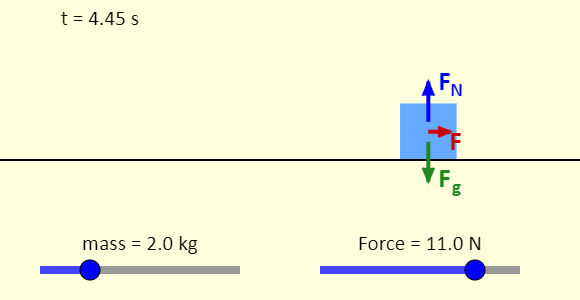This simulation shows the connection between force and motion. A box is placed on a frictionless horizontal surface. You can adjust the mass of the box and the horizontal force applied to the box. Note that the free-body diagram is shown. The blue arrow is the normal force applied to the box by the surface; the green arrow is the force of gravity applied to the box by the Earth, and the red force (if there is one) is the force you control with the slider. Apply a non-zero force to the box and press Play. You should see the box start to move. What happens when you set the force back to zero? How can you make the box stop moving? Force and MotionA Car Race: The cars are of different masses and will be acted on by different forces. Before the race, please guess with good reasons which one will cross the red line first. Assume all tracks are smooth. Motion Under a Player-Controlled Force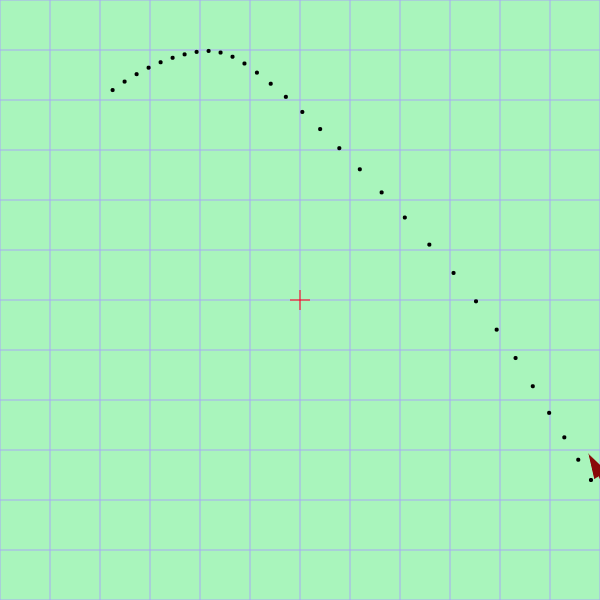Very nice simulation showing the motion of an object under a player-controlled force. This is an excellent simulation for showing how acceleration affects the motions of a moving object. Newton's Third Law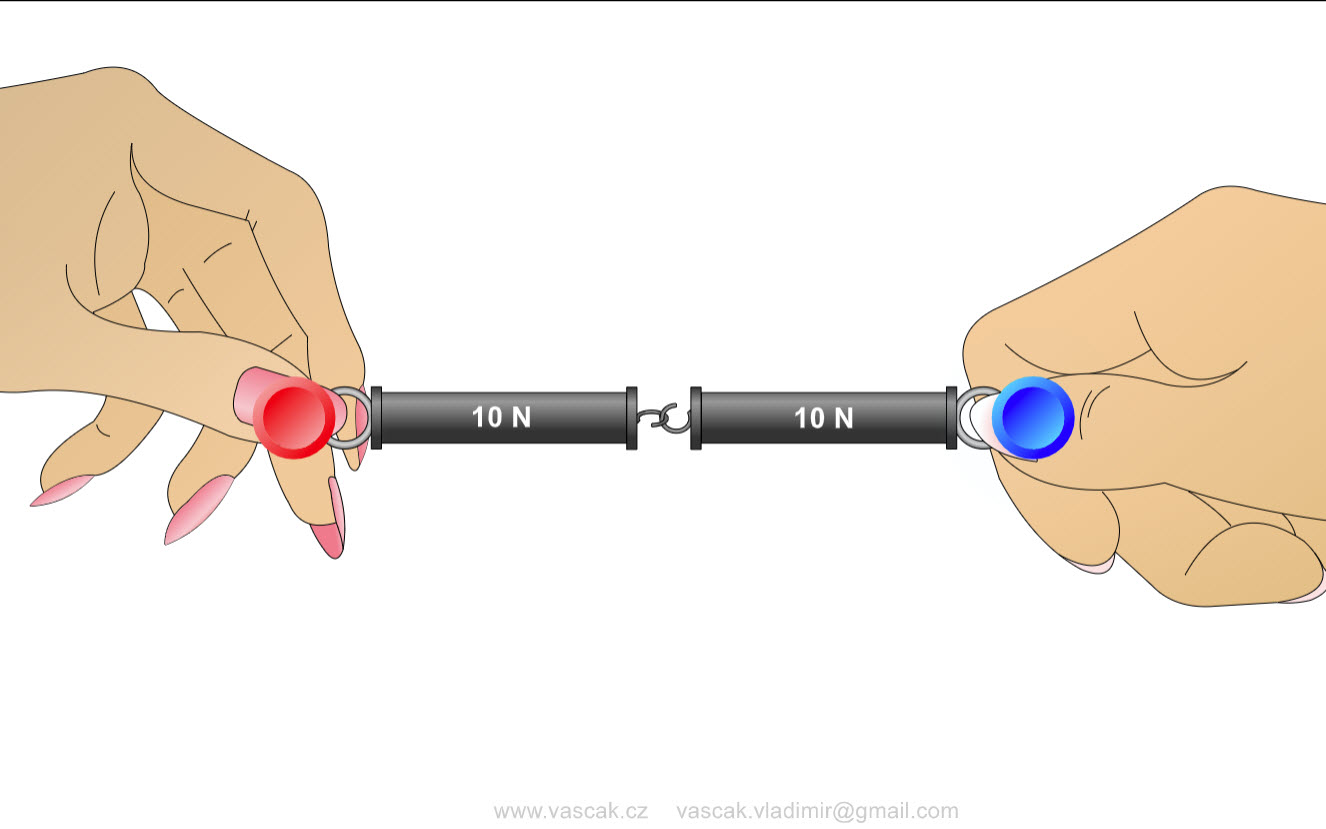This simple animation explores Newton's 3rd Law. Force and Motion: BasicsExplore the forces at work when pulling against a cart, and pushing a refrigerator, crate, or person. Create an applied force and see how it makes objects move. Change friction and see how it affects the motion of objects. Forces and MotionExplore the forces at work when you try to push a filing cabinet. Create an applied force and see the resulting friction force and total force acting on the cabinet. Charts show the forces, position, velocity, and acceleration vs. time. View a Free Body Diagram of all the forces (including gravitational and normal forces). Forces in 1 DimensionExplore the forces at work when you try to push a filing cabinet. Create an applied force and see the resulting friction force and total force acting on the cabinet. Charts show the forces, position, velocity, and acceleration vs. time. View a Free Body Diagram of all the forces (including gravitational and normal forces). Exploring the Normal ForceIn this simulation, you see, on the left, a picture of a box at rest on a table. You can apply a vertical force (up or down) to the box. On the right, you can see the full free-body diagram of the box. The free-body diagram shows the gravitational force (green) exerted on the box by the Earth, the normal force (purple) exerted by the table, and the vertical force (dark blue) that you apply. Note that, on the free-body diagram, all three forces are along the vertical line passing through the center of the box, but the force of gravity and the normal force have been shifted a little so they can be seen more easily. Friction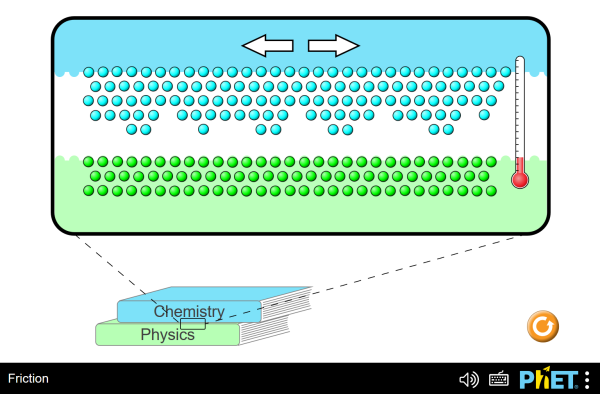Move the Chemistry book and observe what happens. Exploring Static Friction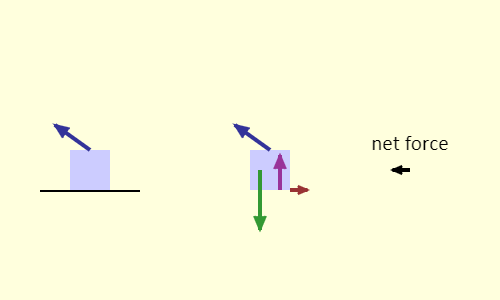In this simulation, you see, on the left, a picture of a box at rest on a table. You can apply a force to the box - note that you set the y-component and the x-component separately. On the right, you can see the full free-body diagram of the box. The free-body diagram shows the gravitational force exerted on the box by the Earth, the normal force exerted by the table, the force that you apply, and, if there is one, the static force of friction. Note that, on the free-body diagram, the force of gravity and the normal force have been shifted horizontally a little so they can be seen more easily. If there is a net force, that is also indicated. Force and Motion with Friction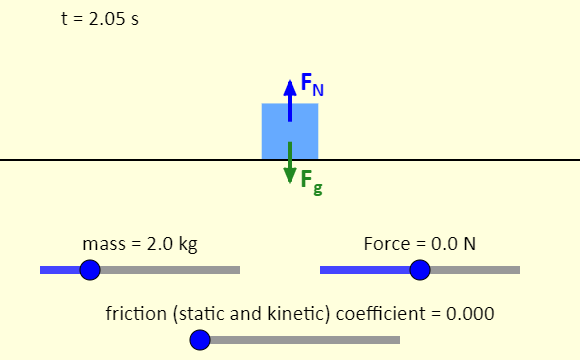This simulation shows the connection between force and motion, with friction. A box is placed on a horizontal surface. You can adjust the mass of the box and the horizontal force applied to the box, as well as the coefficient of friction (the same value for static friction and kinetic friction). Note that the free-body diagram is shown. The blue arrow is the normal force applied to the box by the surface; the green arrow is the force of gravity applied to the box by the Earth, the red force (if there is one) is the force you control with the slider, and the black force (if there is one) is the force of friction. Apply a non-zero force to the box. You should see the box start to move, as long the applied force is larger than the maximum possible force of static friction. How can you cause the box to stop moving? Does everything about the behavior of the force of friction make sense to you? Friction on an Incline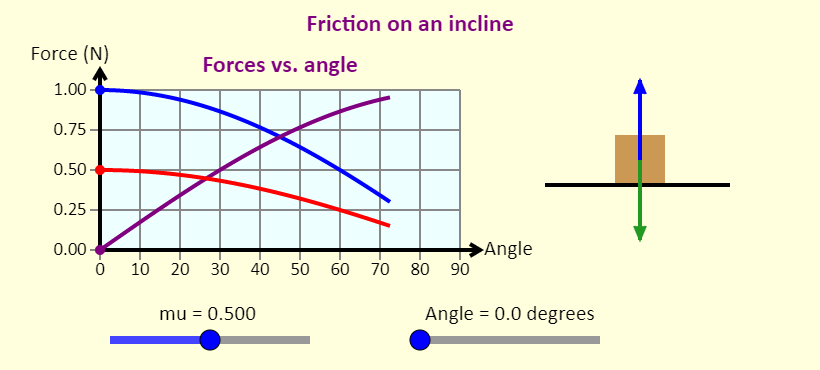Adjust the angle of the incline, and see what happens to the various force components. Note that the slope turns red when there is not enough static friction to keep the block at rest. Can you identify the various arrows on the free-body diagram, and figure out which forces are being plotted on the graph as you adjust the angle? Hooke's Law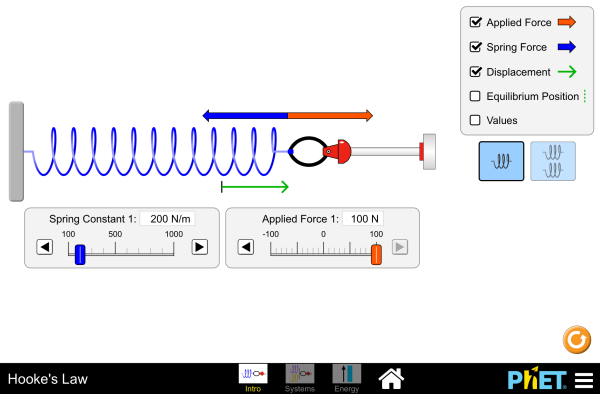Stretch and compress springs to explore the relationships between force, spring constant, displacement, and potential energy! Investigate what happens when two springs are connected in series and parallel. Masses and Springs: Basics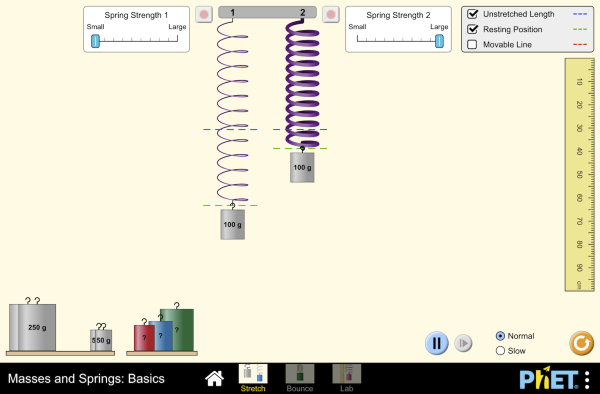Hang masses from springs and discover how they stretch and oscillate. Compare two mass-spring systems, and experiment with spring constant. Transport the lab to different planets, slow down time, and observe the velocity and acceleration throughout the oscillation. Masses and Springs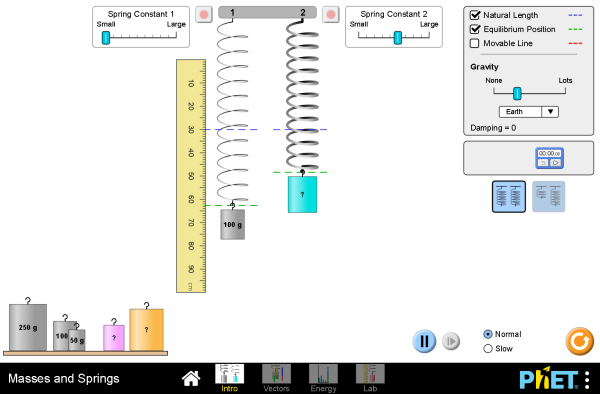Hang masses from springs and adjust the spring constant and damping. Transport the lab to different planets, or slow down time. Observe the forces and energy in the system in real-time, and measure the period using the stopwatch. Gravity Force Lab: Basics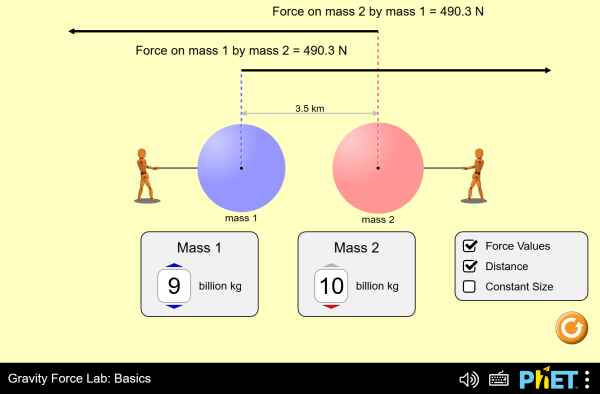Visualize the gravitational force that two objects exert on each other. Discover the factors that affect gravitational attraction, and determine how adjusting these factors will change the gravitational force. Gravity Force Lab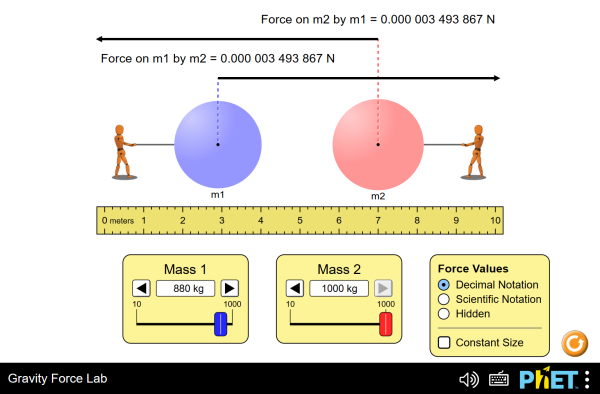Visualize the gravitational force that two objects exert on each other. Adjust properties of the objects to see how changing the properties affects the gravitational attraction. Gravity and Orbits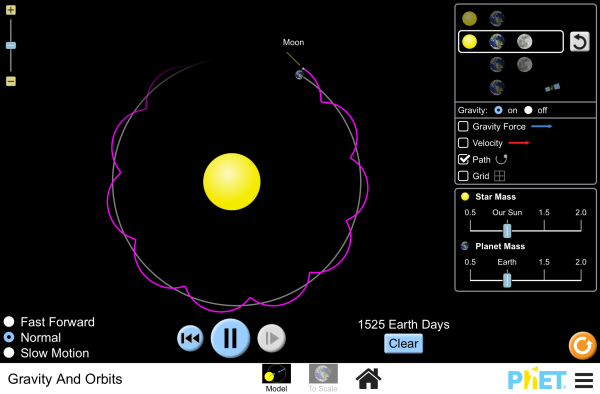Move the sun, earth, moon and space station to see how it affects their gravitational forces and orbital paths. Visualize the sizes and distances between different heavenly bodies, and turn off gravity to see what would happen without it! Ramp: Forces and Motion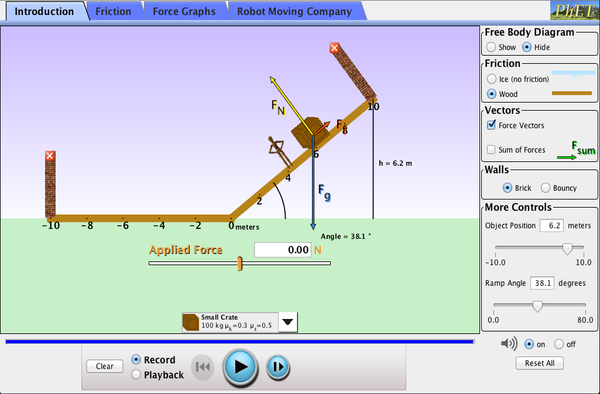Explore forces and motion as you push household objects up and down a ramp. Lower and raise the ramp to see how the angle of inclination affects the parallel forces. Graphs show forces, energy and work. The Ramp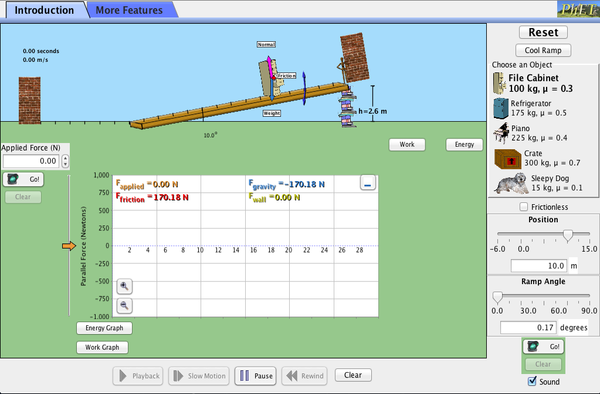Explore forces, energy and work as you push household objects up and down a ramp. Lower and raise the ramp to see how the angle of inclination affects the parallel forces acting on the file cabinet. Graphs show forces, energy and work. Inclined Plane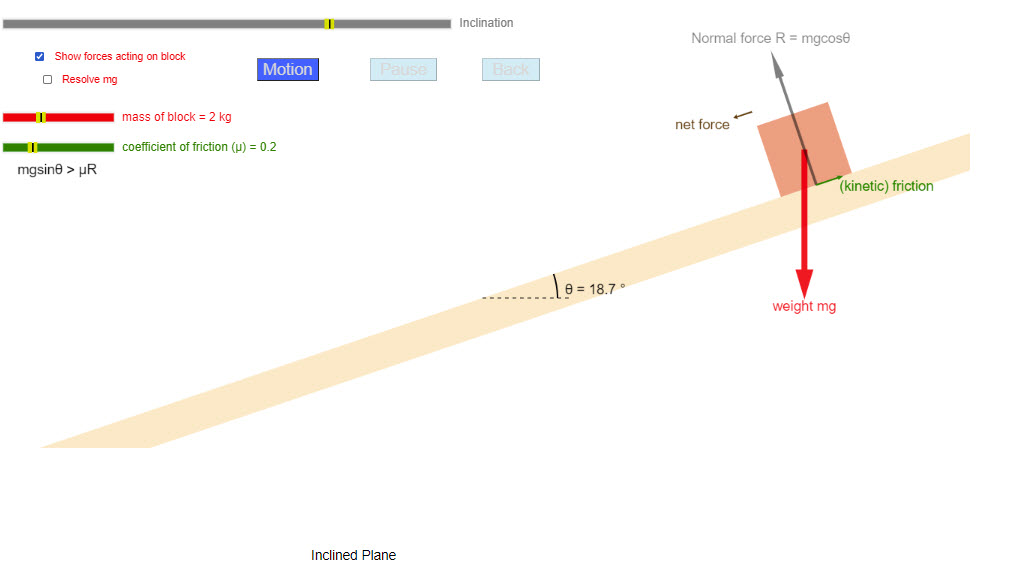Motion of an object on an inclined plane. The user can adjust the angle of the incline, the coefficient of kinectic friction, and the mass of the block. Atwood's Machine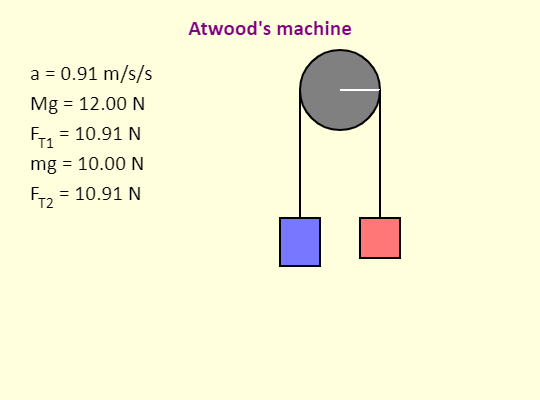The simulation illustrates an Atwood's machine, which is simply two blocks connected by a string passing over a pulley. In this version of the simulation, the mass of the pulley is negligible - that leads to the tension being the same everywhere in the string. Note that the magnitude of the tension is always in between the magnitude of the force of gravity acting on block 1 (blue) and the magnitude of the force of gravity acting on block 2 (red). Given the limits on the sliders in the simulation, and the fact that the simulation uses 10.0 m/s/s for g, what are the most positive and most negative values that can be obtained for the acceleration? Newton's Second Law Experiment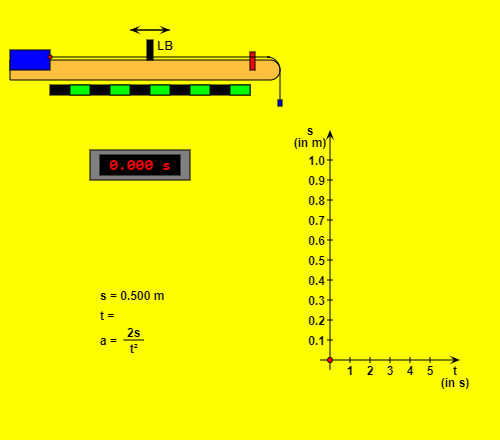This HTML5 app simulates an air track glider setup, as it is used for experiments on constant acceleration motion. A gravitational acceleration of 9.81 m/s2 was presupposed. The mass of the wagon, the value of the hanging mass and the coefficient of friction (within certain limits) can be changed. For one measurement you have to adjust the measuring distance (from the initial position to the light barrier LB, accuracy 5 mm) with pressed mouse button and to read the corresponding time (digital display, accuracy 1 ms). During the movement a red point in the t-s-diagram (time - displacement) indicates the present time and the covered distance. As soon as the measurement of time is finished, the pair of measured values will be marked on the diagram. After a mouse click on the "Record data" button the data will be registered on the list. Motion with Constant Acceleration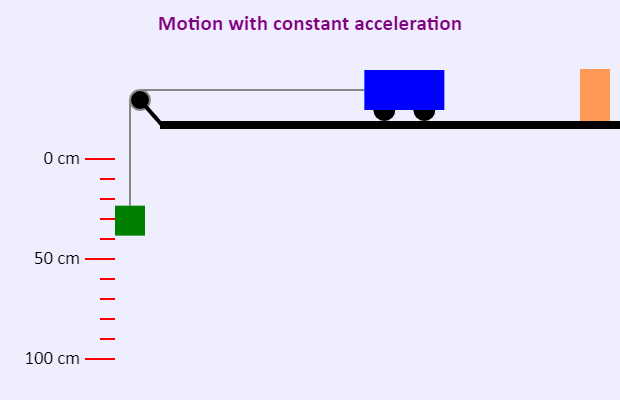This simulation shows a modified Atwood's machine. Model of a Carousel (Centripetal Force)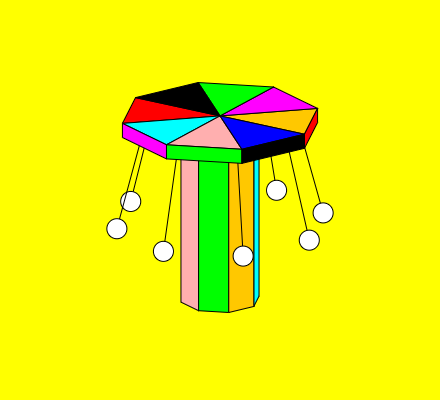The speed of a moving body and its direction of movement remain constant according to Newton's first law (law of inertia) if no force acts on it. The circumstances of a circular motion are different: In this case there must be a force, the so-called centripetal force, which is directed to the axis of rotation. This simplified model of a carousel demonstrates this force.If you choose the second one of the four radio buttons on the top right, the vector arrows of the exerted forces will be drawn for each of the eight pendulum masses: the weight force will be painted black, the force exerted by the string blue. Addition of these vectors results in the net force (red) which is identical with the mentioned inward pointing centripetal force.In addition to the simulation of the carousel (with force arrows or without them) the program offers a simple two-dimensional sketch of the force vectors and important numerical values of the circular motion. If you want to observe exactly the force arrows, you can stop the rotation by using the "Pause / Resume" button or make it ten times slower with the option "Slow motion". The text fields make it possible to vary the parameters within certain limits (don't forget to press the "Enter" key!). Note: The simulation assumes a circular motion with constant angular velocity; the proceedings while the masses are accelerated respectively slowed down are not taken into consideration. The effects of air resistance are neglected, too. Model of a Looping Coaster (Centripetal Force)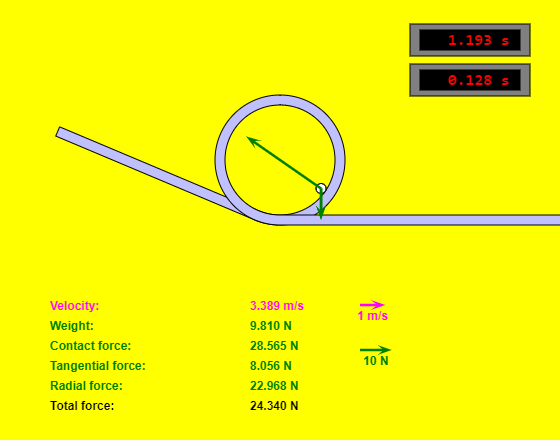A nice simulation that shows the forecs acting on a looping coaster. The user can adjust the initial height, loop radius, mass, and acceleration due to gravity.